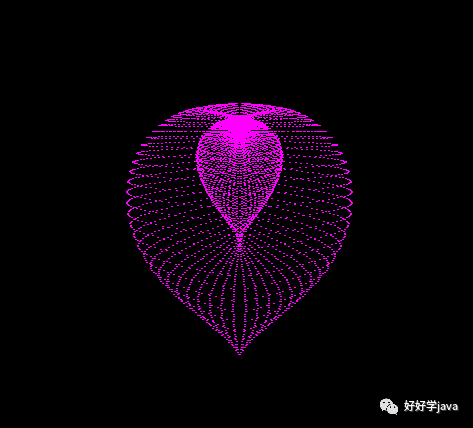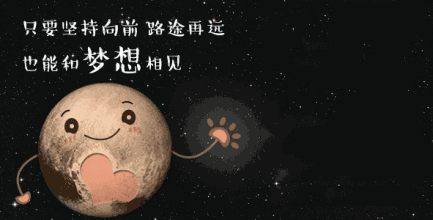java知识分享/学习资源免费分享精彩内容``````
1import java.awt.*;
2import javax.swing.JFrame;
3public class ILOVEYOU extends JFrame {
4    //获取屏幕大小
5    private static final int WIDTH = 500;
6    private static final int HEIGHT = 500;
7    private static int WINDOW_WIDTH = Toolkit.getDefaultToolkit().getScreenSize().width;
8    private static int WINDOW_HEIGHT = Toolkit.getDefaultToolkit().getScreenSize().height;
9    public ILOVEYOU(){
10        super("I love you");//设置窗口标题
11        this.setBackground(Color.BLACK);
12        this.setLocation((WINDOW_WIDTH-WIDTH)/2,(WINDOW_HEIGHT-HEIGHT)/2);//设置窗口位置
13        this.setSize(WIDTH, HEIGHT);//设置窗口大小
14        this.setLayout(getLayout());//设置窗口布局
15        this.setVisible(true);//设置窗口可见
16        this.setDefaultCloseOperation(DISPOSE_ON_CLOSE);//设置窗口默认关闭方式
17    }
18    public void paint(Graphics g){
19        double x,y,r;//横纵坐标以及半径
20        Image image = this.createImage(WIDTH, HEIGHT);
21        Graphics pic = image.getGraphics();
22        for (int i = -2; i &lt; 90; i++) {
23            for (int j = -2; j &lt; 90; j++) {
24                r=Math.PI/45+Math.PI/45*i*(1-Math.sin(Math.PI/45*j))*18;
25                x=r*Math.cos(Math.PI/45*j)*Math.sin(Math.PI/45*i)+WIDTH/2;
26                y=-r*Math.sin(Math.PI/45*j)+HEIGHT/3;
27                pic.setColor(Color.MAGENTA);
28                pic.fillOval((int)x, (int)y, 2, 2);
29            }
30            g.drawImage(image,0,0,this);//生成图片
31        }
32    }
33    public static void main(String[] args) {
34        new ILOVEYOU();
35    }
36}
``````长按二维码关注

上一篇mysql——java.lang.ClassCastException—— java.base,java.math.BigInteger出现类型转换错误

2021-04-04并发基础篇（六）——线程Thread类的start()方法和run()方法

2021-04-04